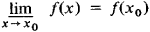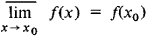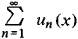# Semicontinuous Function

## Semicontinuous Function

a concept in mathematical analysis. A lower semicontinuous function at a point x0 is a function such thatCorrespondingly for an upper function,In other words, a function is lower semicontinuous at x0 if for every ε > 0 a number δ > 0 can be found such that ǀxx0ǀ < δ implies f(x0) — f(x) < ε (not in absolute value!).

A function that is both upper and lower semicontinuous is continuous in the usual sense. A number of properties of semi-continuous functions are analogous to those of continuous functions. For example, if f(x) and g(x) are lower semicontinuous, their sum and product are also lower semicontinuous; likewise, a lower semicontinuous function on an interval attains its minimum. If the functions un (x), n = 1,2, …, are lower semicontinuous and ≥0, the sumis lower semicontinuous. Semicontinuous functions are Baire functions of class 1.

Mentioned in ?
References in periodicals archive ?
Recall the proximal mapping of a proper, convex and lower semicontinuous function g : C [right arrow] R with a parameter [lambda] > 0 as follows:
Among those generalizations, there is Kirk-Saliga fixed point theorem (see ) which states that any map T : X [right arrow] X has a fixed point provided that X is complete metric space and there exist an integer p [member of] N and a lower semicontinuous function [phi] : X [right arrow] [0, [infinity]) such that
Let j : R [right arrow] (-[infinity], [infinity]] be proper, convex, and lower semicontinuous function, p [member of] [2, [infinity]) and [lambda] > 0.
with gx [less than or equal to] gu and gv [less than or equal to] gy, or gu [less than or equal to] gx and gy [less than or equal to] gv for all x, y, u, v [member of] X, F [member of] F, ([psi], [phi]) [member of] [PSI], [psi] is nondecreasing, and [phi] : X [right arrow] [0, +[infinity]) is a lower semicontinuous function.
Let [K.sub.0] be a compact set in E and let g(*) : E [right arrow] R be a lower semicontinuous function. If Q : PC([0, b], E) [right arrow] [L.sup.p] ([0, b], E) is a causal operator such that the condition ([H.sub.1])-([H.sub.3]) hold, then the control problem (3.1) has an optimal solution; that is, there exists [[xi].sub.0] [member of] [K.sub.0] and [u.sub.0] (*) [member of] [S.sub.T] ([[xi].sub.0]) such that g([u.sub.0] (T)) = inf (g(u(T)); u(*) [member of] [S.sub.T] ([[xi].sub.0]), [[xi].sub.0] [member of] [K.sub.0]}.
Let [eta] : K x K [right arrow] R [union] {+[infinity]} be a proper convex lower semicontinuous function and [eta] : K x K [right arrow] E be a mapping.
Let f: X [right arrow] R [union]{+[infinity]} be a proper lower semicontinuous function. For -[infinity] < [[eta].sub.1] < [[eta].sub.2] [less than or equal to] +[infinity], let us set [[[eta].sub.1] < f < [[eta].sub.2]] = {x [member of] X : 1 < f(x) < 2}.
Hence, f is a soft semicontinuous function but not soft [alpha]-continuous function.
If in Theorem 1 A is the subdifferential of a proper, convex and lower semicontinuous function [phi] : H [right arrow] (-[infinity], +[infinity]] and F is nonempty (i.e., [phi] has at least a minimum point), then it is easy to see that
([P.sup.2]) for fixed u, it is an upper semicontinuous function of [MATHEMATICAL EXPRESSION NOT REPRODUCIBLE IN ASCII].
Suppose that f is a continuous function on a neighborhood of x and [f'.sub.+] is finite on a neighborhood (x, v).Then [f'.sub.+] is a upper semicontinuous function at point (x, v).

Site: Follow: Share:
Open / Close